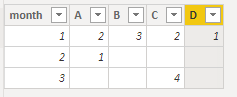cancel
Showing results for
Did you mean:Anonymous
Not applicable

## How to creat a new table have all of columns from the value of a column of another table

Hi Eveyon

I am new and i speak english not well.

Pls help me for this case:

I have table name: "NL_TH"So, I want creat new table as belowI cannot user pivot and unpivot because Table :NL_TH is not original data .

Thank for help

1 ACCEPTED SOLUTIONCommunity Support

Hi  @Anonymous ,

Here are the steps you can follow：

1. Create calculated table.

``````Table =
SUMMARIZE('NL_TH',NL_TH[month])``````

2. Create calculated column.

``````A =
CALCULATE(SUM('NL_TH'[value]),FILTER('NL_TH','NL_TH'[DONVI]="A"&&'NL_TH'[month]=EARLIER('Table'[month])))``````
``````B =
CALCULATE(SUM('NL_TH'[value]),FILTER('NL_TH','NL_TH'[DONVI]="B"&&'NL_TH'[month]=EARLIER('Table'[month])))``````
``C = CALCULATE(SUM('NL_TH'[value]),FILTER('NL_TH','NL_TH'[DONVI]="C"&&'NL_TH'[month]=EARLIER('Table'[month])))``
``D = CALCULATE(SUM('NL_TH'[value]),FILTER('NL_TH','NL_TH'[DONVI]="D"&&'NL_TH'[month]=EARLIER('Table'[month])))``

3. Result:Best Regards,

Liu Yang

If this post helps, then please consider Accept it as the solution to help the other members find it more quickly.

3 REPLIES 3Anonymous
Not applicable

Thanks for helpCommunity Support

Hi  @Anonymous ,

Here are the steps you can follow：

1. Create calculated table.

``````Table =
SUMMARIZE('NL_TH',NL_TH[month])``````

2. Create calculated column.

``````A =
CALCULATE(SUM('NL_TH'[value]),FILTER('NL_TH','NL_TH'[DONVI]="A"&&'NL_TH'[month]=EARLIER('Table'[month])))``````
``````B =
CALCULATE(SUM('NL_TH'[value]),FILTER('NL_TH','NL_TH'[DONVI]="B"&&'NL_TH'[month]=EARLIER('Table'[month])))``````
``C = CALCULATE(SUM('NL_TH'[value]),FILTER('NL_TH','NL_TH'[DONVI]="C"&&'NL_TH'[month]=EARLIER('Table'[month])))``
``D = CALCULATE(SUM('NL_TH'[value]),FILTER('NL_TH','NL_TH'[DONVI]="D"&&'NL_TH'[month]=EARLIER('Table'[month])))``

3. Result:Best Regards,

Liu Yang

If this post helps, then please consider Accept it as the solution to help the other members find it more quickly.Super User

@Anonymous

have you tried to use matrix to display this?Proud to be a Super User!